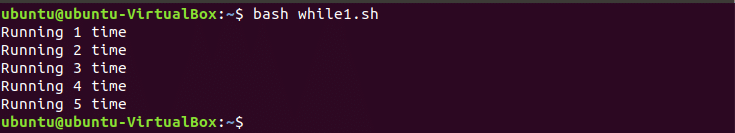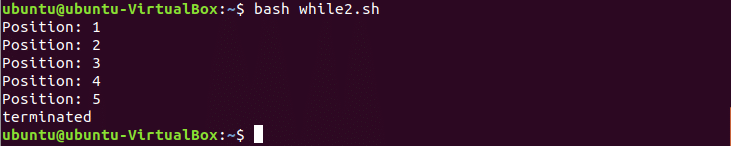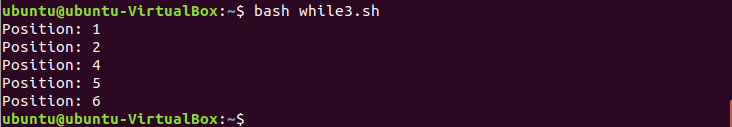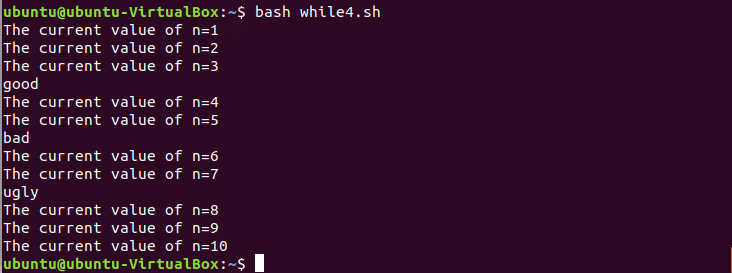BASH Programming

# BASH While Loop Examples

Three types of loops are used in bash programming. While loop is one of them. Like other loops, while loop is used to do repetitive tasks. How you can use while loop in bash script is shown in this article by using different examples.

## Syntax of while loop:

while [ condition ]
do
commands
done

The starting and ending block of while loop are defined by do and done keywords in bash script. Termination condition is defined at the starting of the loop. Open a text editor to write bash script and test the following while loop examples.

### Example-1: Iterate the loop for fixed number of times

Create a bash file named while1.sh which contains the following script.

n=1
while [ \$n -le 5 ]
do
echo "Running \$n time"
(( n++ ))
done

In this example, the loop will iterate for 5 times and print the text which is defined inside the loop. The following output will appear if you run while1.sh.### Example-2: Using break statement for conditional exit

break statement is used to exit from the loop early based on a particular condition. Create a new bash file named while2.sh with the following code.

n=1
while [ \$n -le 10 ]
do
if [ \$n == 6 ]
then
echo "terminated"
break
fi
echo "Position: \$n"
(( n++ ))
done

In this example, the loop is declared to iterate for 10 times.  According to the script it will terminate after 6 times iteration for break statement. The following output will appear after executing the script.### Example-3: Using continue statement to omit particular step

Create a new bash file named while3.sh with the following code.

n=0
while [ \$n -le 5 ]
do
(( n++ ))

if [ \$n == 3 ]
then
continue
fi
echo "Position: \$n"

done

In this example, the loop will iterate for 5 times but it will not print all 5 positions. When the loop will iterate for 3rd times then continue statement will be executed and the loop will go for the next iteration without printing the text of 3rd position. The following output will appear after executing the script.### Example-4: Creating infinite loop

Sometimes, it is required to declare infinite loop for various programming purposes. Create a new bash file named while4.sh and test the code of infinite loop.

n=1
while :
do
printf "The current value of n=\$n\n"
if [ \$n == 3 ]
then
echo "good"
elif [ \$n == 5 ]
then
elif [ \$n == 7 ]
then
echo "ugly"
elif [ \$n == 10 ]
then
exit 0
fi
((n++))
doneNo termination condition is set for the loop in this example. This type of loop is called infinite loop. Here, exit statement is used to quit from the infinite loop. So, this loop will iterated for 10 times and when the iteration value become equal to 10 then exit statement will execute for exiting from the infinite loop.